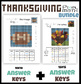# Pixel Thanksgiving Bundle - 10x10 & 15x15 grids for decimals, fractions, percentSubject
Resource Type
File Type

Zip

(19 MB|14 pages)
Product Rating
Standards
2 Products in this Bundle
2 products
1. Math activity sheets based on a pixelated footballEngage your students this Thanksgiving season with (American) Football Pixel Art, a creative way to review and reinforce fluency with decimals, fractions and percents PDF Worksheets Include: Color in the ordered pairs (x,y) to make the shape of a foo
2. Math activity sheets based on a pixelated turkey - 15x15, and 30x30 gridsEngage your students with Turkey Pixel Art, a creative way to challenge students who have already used a 10x10 grid to explore decimals, fractions and percentsPDF Worksheets Include: Color in the ordered pairs (x,y) to make the
• Bundle Description
• StandardsNEW

Individual sets (10x10 Football and 15x15 Turkey) available separately for \$3 each

At least three activities in each set (coloring in the ordered pairs, analyzing the squares and magnifying the image) as well as blank sheets for unlimited variations when students/teacher make and analyze their own images

Set One

Math activity sheets based on a pixelated football (10x10 grid)

PDF Worksheets:

• Color in the ordered pairs (x,y) to make the shape of a football
• Analyze the shaded squares to find number of blue/brown/tan squares and write fraction, decimal, and percent
• Magnify the image from a 10x10 grid to a 20x20 grid

also includes

• Answer Key with the tables filled in
• Blank 10x10 grid for students to create and analyze their own Fall/Sport/Thanksgiving image
• Blank 20x20 grid for students to create and analyze their own Fall/Sport/Thanksgiving image

Worksheets are self checking, in that all answers must add up = to 100 squares (400 squares for 20x20 grid), 1.00 decimals, 100/100 fractions (or 400/400 for 20x20 grid) and 100%

Set Two

Math activity sheets based on a pixelated turkey (15x15 grid)

PDF Worksheets:

• Color in the ordered pairs (x,y) to make the shape of a pixelated turkey
• Analyze the shaded squares to find number of yellow/black/blue/brown/red/ orange/green squares and write fraction, decimal, and percent
• Magnify the image from a 15x15 grid to a 30x30 grid
• Process requires rounding to the nearest thousandth/hundredth at different stages - prompts on worksheet remind students of this, but further instruction may be helpful

also includes

• Answer Key with the tables filled in
• Blank 15x15 grid for students to create and analyze their own Thanksgiving image
• Blank 30x30 grid for students to create and analyze their own Thanksgiving image

Worksheets are self checking, in that all answers must add up = to 225 squares (900 squares for 30x30 grid), 1.00 decimals, 225/225 fractions (or 900/900 for 30x30 grid) and 100%

Use decimal notation for fractions with denominators 10 or 100. For example, rewrite 0.62 as 62/100; describe a length as 0.62 meters; locate 0.62 on a number line diagram.
Express a fraction with denominator 10 as an equivalent fraction with denominator 100, and use this technique to add two fractions with respective denominators 10 and 100. For example, express 3/10 as 30/100, and add 3/10 + 4/100 = 34/100.
Interpret a fraction as division of the numerator by the denominator (𝘢/𝘣 = 𝘢 ÷ 𝘣). Solve word problems involving division of whole numbers leading to answers in the form of fractions or mixed numbers, e.g., by using visual fraction models or equations to represent the problem. For example, interpret 3/4 as the result of dividing 3 by 4, noting that 3/4 multiplied by 4 equals 3, and that when 3 wholes are shared equally among 4 people each person has a share of size 3/4. If 9 people want to share a 50-pound sack of rice equally by weight, how many pounds of rice should each person get? Between what two whole numbers does your answer lie?
Compare two fractions with different numerators and different denominators, e.g., by creating common denominators or numerators, or by comparing to a benchmark fraction such as 1/2. Recognize that comparisons are valid only when the two fractions refer to the same whole. Record the results of comparisons with symbols >, =, or <, and justify the conclusions, e.g., by using a visual fraction model.
Explain why a fraction 𝘢/𝘣 is equivalent to a fraction (𝘯 × 𝘢)/(𝘯 × 𝘣) by using visual fraction models, with attention to how the number and size of the parts differ even though the two fractions themselves are the same size. Use this principle to recognize and generate equivalent fractions.
Total Pages
14 pages
Included
Teaching Duration
N/A
Report this Resource to TpT
Reported resources will be reviewed by our team. Report this resource to let us know if this resource violates TpT’s content guidelines.